Courses

# Test: Data Handling - 2

## 20 Questions MCQ Test Mathematics (Maths) Class 7 | Test: Data Handling - 2

Description
This mock test of Test: Data Handling - 2 for Class 7 helps you for every Class 7 entrance exam. This contains 20 Multiple Choice Questions for Class 7 Test: Data Handling - 2 (mcq) to study with solutions a complete question bank. The solved questions answers in this Test: Data Handling - 2 quiz give you a good mix of easy questions and tough questions. Class 7 students definitely take this Test: Data Handling - 2 exercise for a better result in the exam. You can find other Test: Data Handling - 2 extra questions, long questions & short questions for Class 7 on EduRev as well by searching above.
QUESTION: 1

Solution:
QUESTION: 2

Solution:
QUESTION: 3

### Which class has the lowest frequency?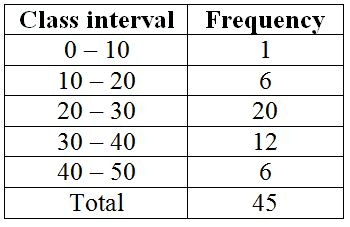Solution:
QUESTION: 4

What is the lower limit of the class interval 40 – 50?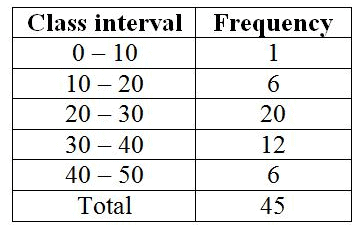Solution:

Lower limit is the least limit of the class interval. So its 40

QUESTION: 5

What is the size of the class interval?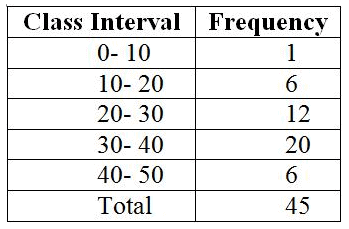Solution:
QUESTION: 6

Which class has the highest frequency?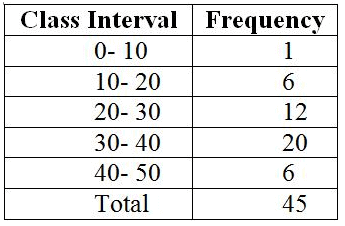Solution:
QUESTION: 7

When a die is thrown, total number of possible outcomes is ______.

Solution:
QUESTION: 8

When a coin is thrown, total number of possible outcomes is ______.

Solution:
QUESTION: 9

Two dice are thrown, find and number of outcomes.

Solution:

Since two dice are thrown simultaneously total numbers of outcomes are 6*6=36 . We make pairs of it
(1,1)   (2,1)   (3,1).....(6,1)
:         :         :    :::::     :
(1,6)   (2,6)   (3,6).....(6,6)

QUESTION: 10

There are 2 red, 3 blue and 5 black balls in a bag. A ball is drawn from the bag without looking in to the bag. What is the probability of getting a non-red ball?

Solution:
QUESTION: 11

There are 2 red, 3 blue and 5 black balls in a bag. A ball is drawn from the bag without looking in to the bag. What is the probability of getting a red ball?

Solution:

Total number of outcomes = 10
No. of favourable outcomes = 2 red balls
Probability of red balls =  No. of favourable outcomes/Total number of outcomes
= 2/10= 1/5

QUESTION: 12

There are 2 red, 3 blue and 5 black balls in a bag. A ball is drawn from the bag without looking in to the bag. What is the probability of getting a blue ball?

Solution:
QUESTION: 13

There are 2 red, 3 blue and 5 black balls in a bag. A ball is drawn from the bag without looking in to the bag. What is the probability of getting a black ball?

Solution:
QUESTION: 14

There are 2 red, 3 blue and 5 black balls in a bag. A ball is drawn from the bag without looking in to the bag. What is the probability of getting a non-blue ball?

Solution:
QUESTION: 15

There are 2 red, 3 blue and 5 black balls in a bag. A ball is drawn from the bag without looking in to the bag. What is the probability of getting a non-black ball?

Solution:

The number of black balls =5

Probability of getting black ball = 5/10 = 1/2

Probability of getting a non black ball = 1 - Probability of getting a black ball

= 1 -1/2 = 1/2

QUESTION: 16

What is the size of class intervals?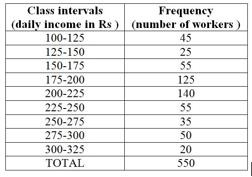Solution:
QUESTION: 17

Which class has the highest frequency?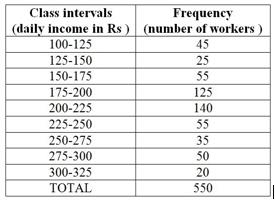Solution:
QUESTION: 18

Which class has the lowest frequency?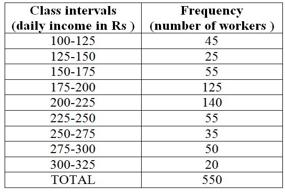Solution:
QUESTION: 19

What is the upper limit of the class interval 250-275?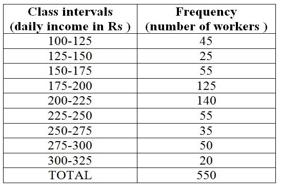Solution:

Upper limit is the largest quantity in the interval. So the upper limit is 275.

QUESTION: 20

What is the frequency of class interval 300-325?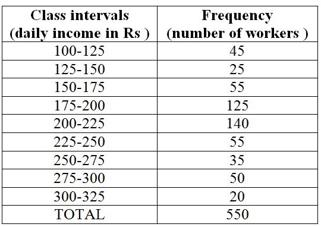Solution: